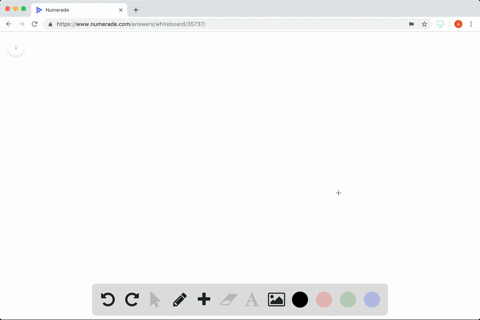### True or False? In Exercises $73-78$ , determine w…

02:56University of Delaware

This question is in the process of being solved. The video shown is an answer to a question that covers similar topics.

Problem 77

True or False? In Exercises $73-78$ , determine whether the statement is true or false. If it is false, explain why or give an example that shows it is false.
$$f^{\prime}(x)=g(x), then \int g(x) d x=f(x)+C$$

True

## Discussion

You must be signed in to discuss.

## Video Transcript

No transcript available